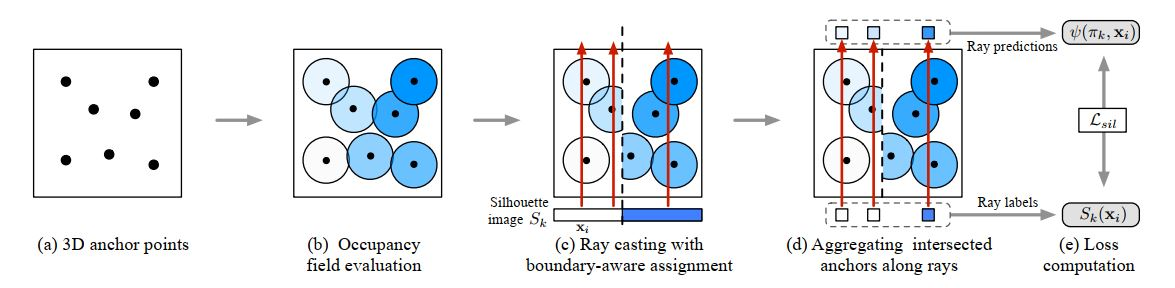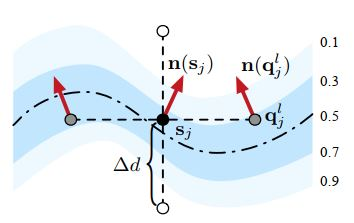NeurIPS2019 Learning of Implicit Surfaces without 3D supervision

## 参考论文

Learning to Infer Implicit Surfaces without 3D Supervision

## Introduction

1. 提出了首个能够不使用3D信息进行监督学习Implicit Surfaces的框架

2. 提出了基于锚点以及探查射线的探查方法有效地将Implicit field与原始二维图像联系起来。

3. 提出了一种有效地取样方法用于implicit surface的生成。

4. 提出了一种通用的几何正则化项用于约束Implicit surface的几何特征。

## Method

### Overview### Network Architecture### Sampling-Based 2D Supervision

$\psi(\pi_k, x_i) = \mathcal{G}(\{\phi(c + r(\pi_k, x_i) \cdot t_j)\}_{j=1}^{N_p})$

$\mathcal{L}_{sil} = \frac{1}{N_r}\sum_{i=1}^{N_r}\sum_{k=1}^{N_K}||\psi(\pi_k, x_i) - S_k(x_i)||^2$

#### Importance Sampling

$\left\{ \begin{array}{ll} P_r(x) = \int_{x'}\kappa(x',x;\sigma)W_r(x')dx' \\ P_p(p) = \int_{p'}\kappa(p',p;\sigma)W_r(p')dp' \end{array} \right.$

### Geometric Regularizaiton on Implicit Surface

$\frac{\delta^n \phi}{\delta p^n_j} = \frac{1}{\Delta d^n}\sum_{l=0}^{n}(-1)^l\binom{n}{l}\phi(p_j + (\frac{n}{2} - l)\Delta d)$

$\mathcal{L}_{geo} = \frac{1}{N_p}\sum_{j=1}^{N_p}W(\phi(s_j))\frac{\sum_{l=1}^6W(\phi(q_j^l))||n(s_j) - n(q_j^l)||^p_p}{\sum_{l=1}^6W(\phi(q_j^l))}$$\mathcal{L} = \mathcal{L}_{sil} + \lambda\mathcal{L}_{geo}$